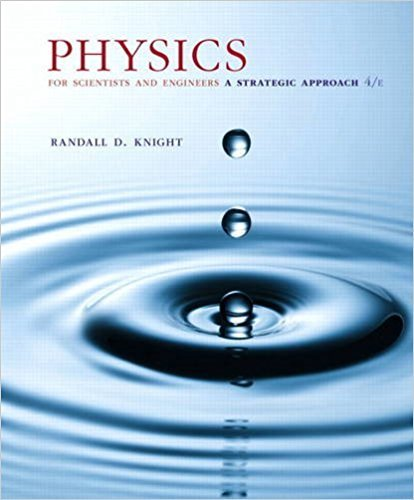# Suppose you have a machine that gives you pieces of candy## Problem 41.89 Chapter 41

Physics for Scientists and Engineers: A Strategic Approach, Standard Edition (Chs 1-36) | 4th Edition

• 2901 Step-by-step solutions solved by professors and subject experts
• Get 24/7 help from StudySoup virtual teaching assistantsPhysics for Scientists and Engineers: A Strategic Approach, Standard Edition (Chs 1-36) | 4th Edition

4 5 0 377 Reviews
16
2
Problem 41.89

Suppose you have a machine that gives you pieces of candy when you push a button. Eighty percent of the time, pushing the button gets you two pieces of candy. Twenty percent of the time, pushing the button yields 10 pieces. The average number of pieces per push is Navg = 2 * 0.80 + 10 * 0.20 = 3.6. That is, 10 pushes should get you, on average, 36 pieces. Mathematically, the average value when the probabilities differ is Navg = g1Ni * Probability of i2. We can do the same thing in quantum mechanics, with the difference that the sum becomes an integral. If you measured the distance of the electron from the proton in many hydrogen atoms, you would get many values, as indicated by the radial probability density. But the average value of r would be ravg = 3 0 rPr1r2 dr Calculate the average value of r in terms of aB for the electron in the 1s and the 2p states of hydrogen.

Step-by-Step Solution:
Step 1 of 3
Step 2 of 3

Step 3 of 3

##### ISBN: 9780134081496

This full solution covers the following key subjects: . This expansive textbook survival guide covers 42 chapters, and 4463 solutions. Physics for Scientists and Engineers: A Strategic Approach, Standard Edition (Chs 1-36) was written by and is associated to the ISBN: 9780134081496. The answer to “Suppose you have a machine that gives you pieces of candy when you push a button. Eighty percent of the time, pushing the button gets you two pieces of candy. Twenty percent of the time, pushing the button yields 10 pieces. The average number of pieces per push is Navg = 2 * 0.80 + 10 * 0.20 = 3.6. That is, 10 pushes should get you, on average, 36 pieces. Mathematically, the average value when the probabilities differ is Navg = g1Ni * Probability of i2. We can do the same thing in quantum mechanics, with the difference that the sum becomes an integral. If you measured the distance of the electron from the proton in many hydrogen atoms, you would get many values, as indicated by the radial probability density. But the average value of r would be ravg = 3 0 rPr1r2 dr Calculate the average value of r in terms of aB for the electron in the 1s and the 2p states of hydrogen.” is broken down into a number of easy to follow steps, and 168 words. Since the solution to 41.89 from 41 chapter was answered, more than 228 students have viewed the full step-by-step answer. The full step-by-step solution to problem: 41.89 from chapter: 41 was answered by , our top Physics solution expert on 12/28/17, 08:06PM. This textbook survival guide was created for the textbook: Physics for Scientists and Engineers: A Strategic Approach, Standard Edition (Chs 1-36), edition: 4.

Unlock Textbook Solution

Suppose you have a machine that gives you pieces of candy

×
Get Full Access to Physics For Scientists And Engineers: A Strategic Approach, Standard Edition (Chs 1 36) - 4 Edition - Chapter 41 - Problem 41.89

Get Full Access to Physics For Scientists And Engineers: A Strategic Approach, Standard Edition (Chs 1 36) - 4 Edition - Chapter 41 - Problem 41.89

I don't want to reset my password

Need help? Contact support

Need an Account? Is not associated with an account
We're here to help